# Some simple examples of manipulating the axes in a graph

## 2014-01-01

I want to start with a simple artificial example, a cubic function. With this simple function, you will see different ways of displaying information on the x and y axes.

x <- seq(-2,2,length=1000)
y <- x^3-2*x
plot(x,y)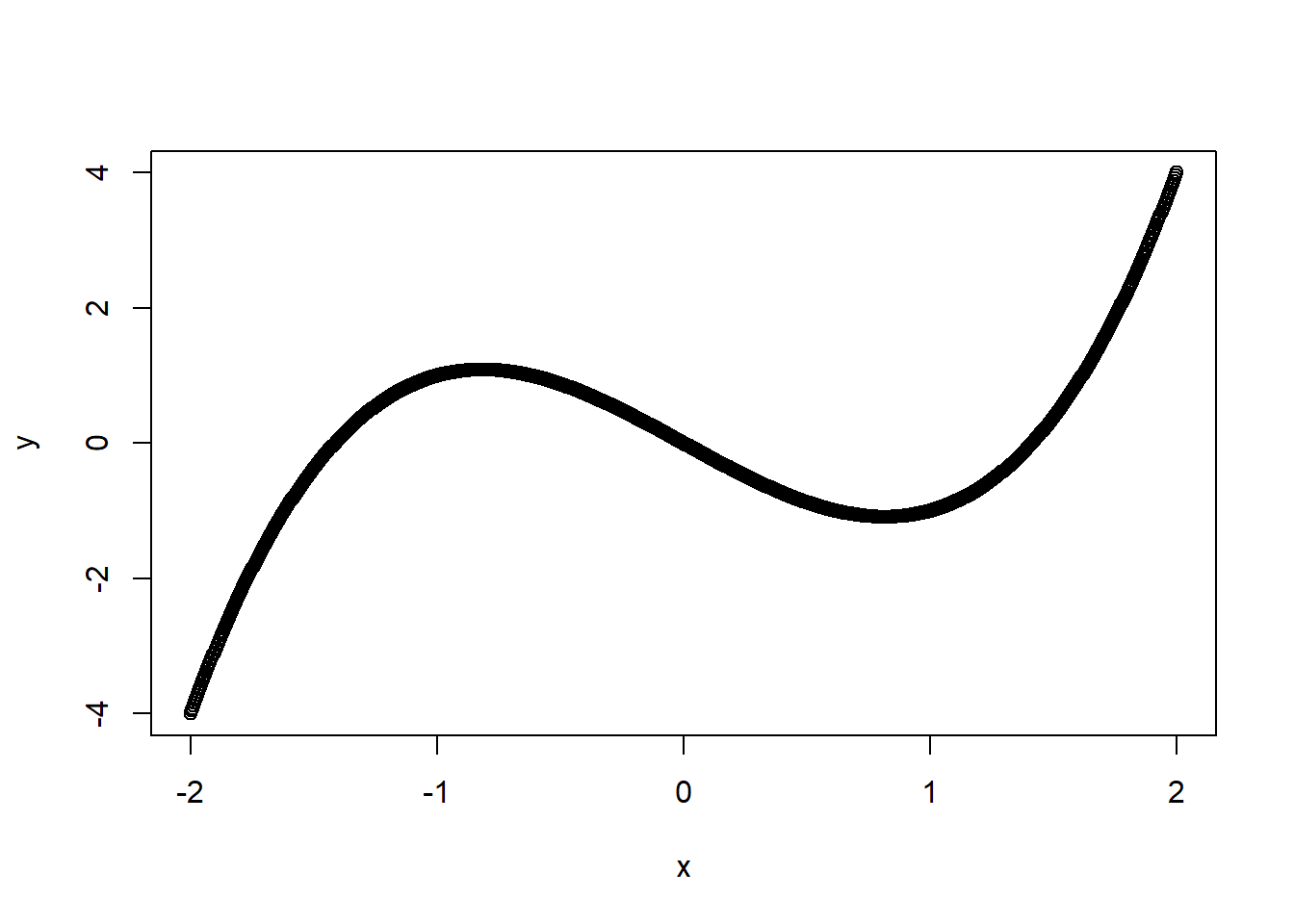Figure 1. Plot of a simple cubic function.

This is a classic cubic function with a local minimum at sqrt(2/3), local maximum at -sqrt(2/3) and zeros at -sqrt(2), 0, and +sqrt(2). Let’s draw the tick marks at these locations instead.

xticks <- c(-sqrt(2),-sqrt(2/3),0,sqrt(2/3),sqrt(2))
plot(x,y,axes=FALSE)
axis(side=2)
axis(side=1,at=xticks)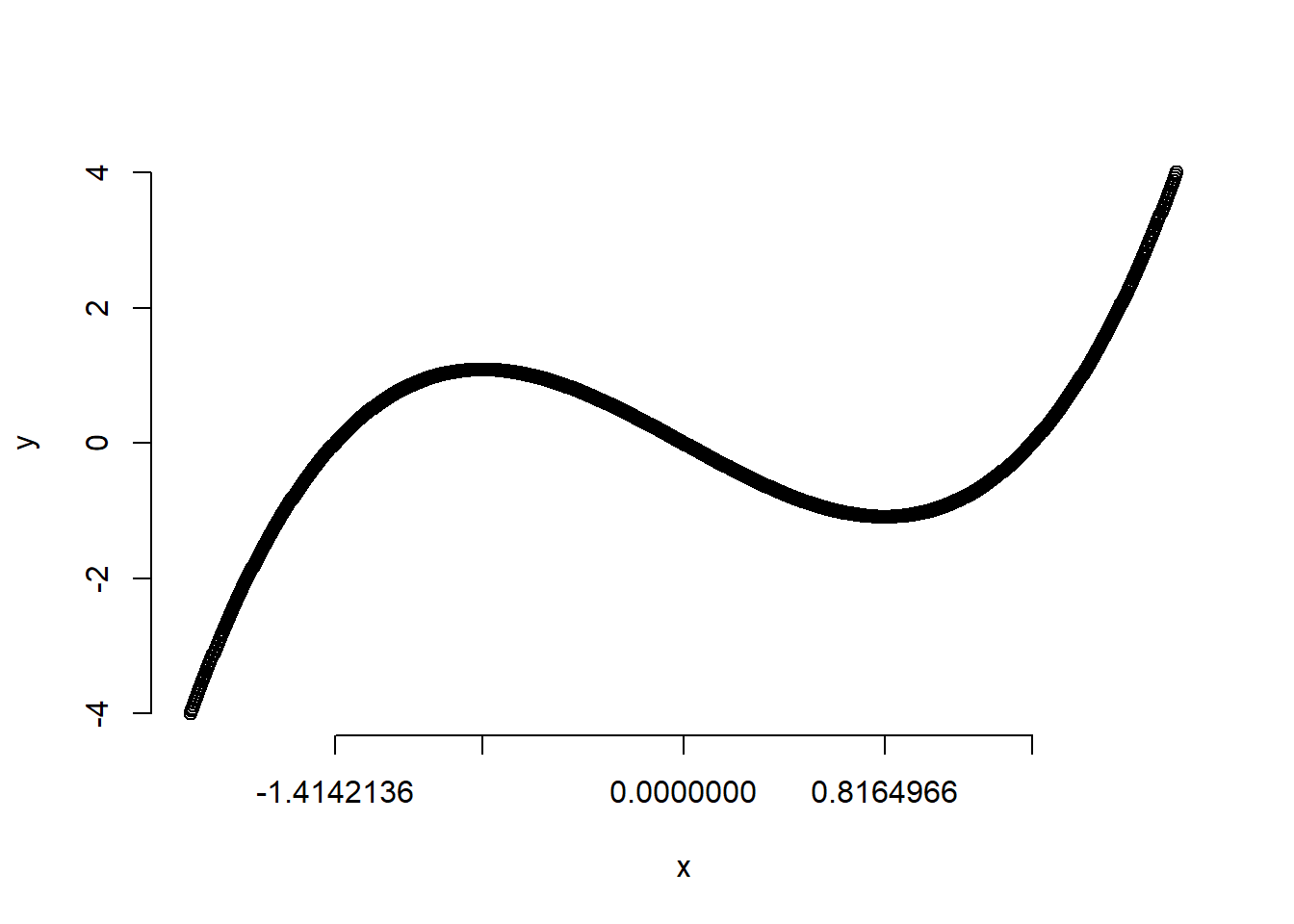Figure 2. Plot with different tick locations.

Let’s make the labels fit better.

plot(x,y,axes=FALSE)
axis(side=2)
axis(side=1,at=xticks,labels=round(xticks,3))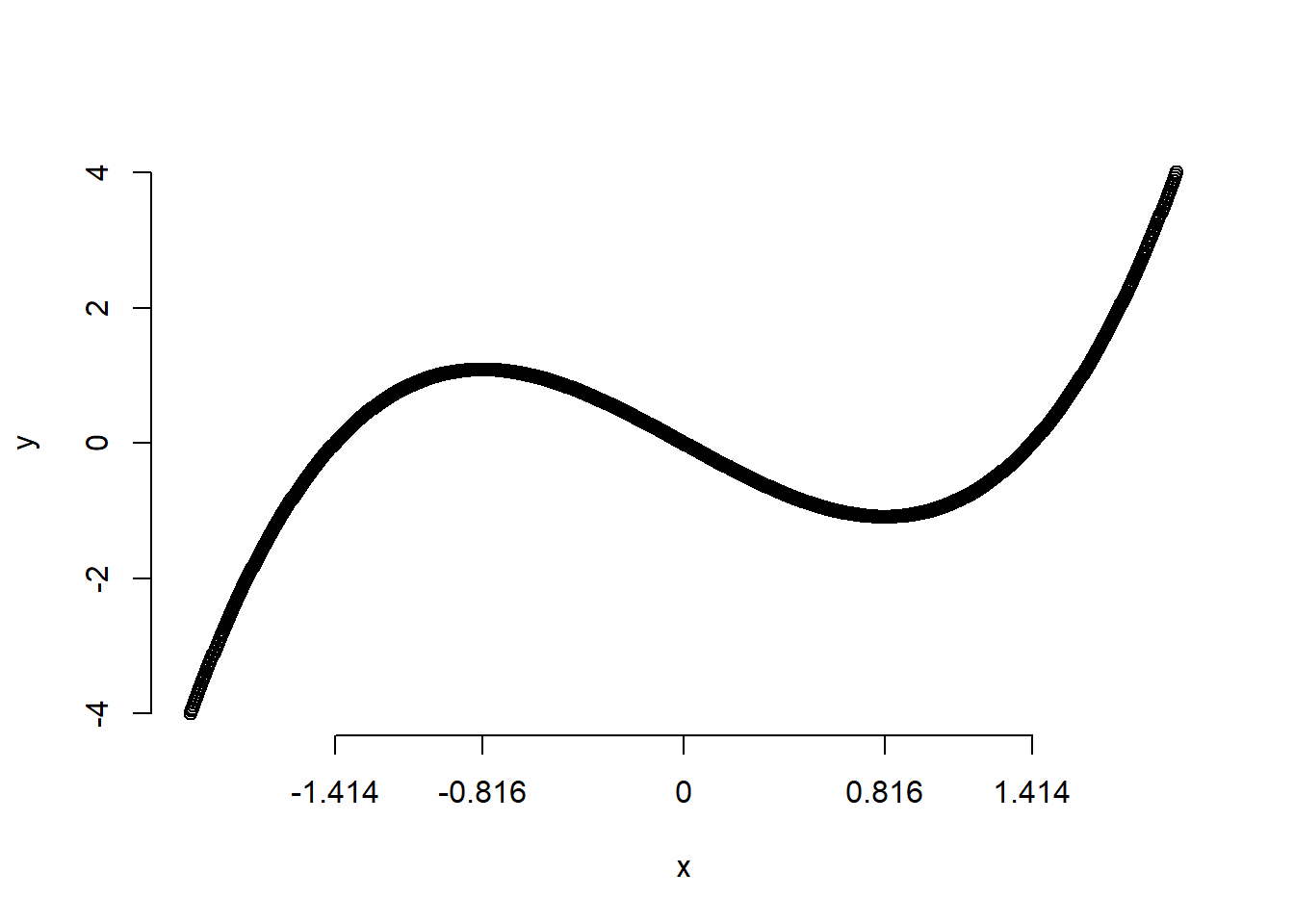Figure 3. Plot with rounded values at tick marks.

If you like, you can use math expressions on your axes

plot(x,y,axes=FALSE)
xlabs <- c(expression(-sqrt(2)),expression(-sqrt(2/3)),expression(0),expression(sqrt(2/3)),expression(+sqrt(2)))
axis(side=2)
axis(side=1,at=xticks,labels=xlabs)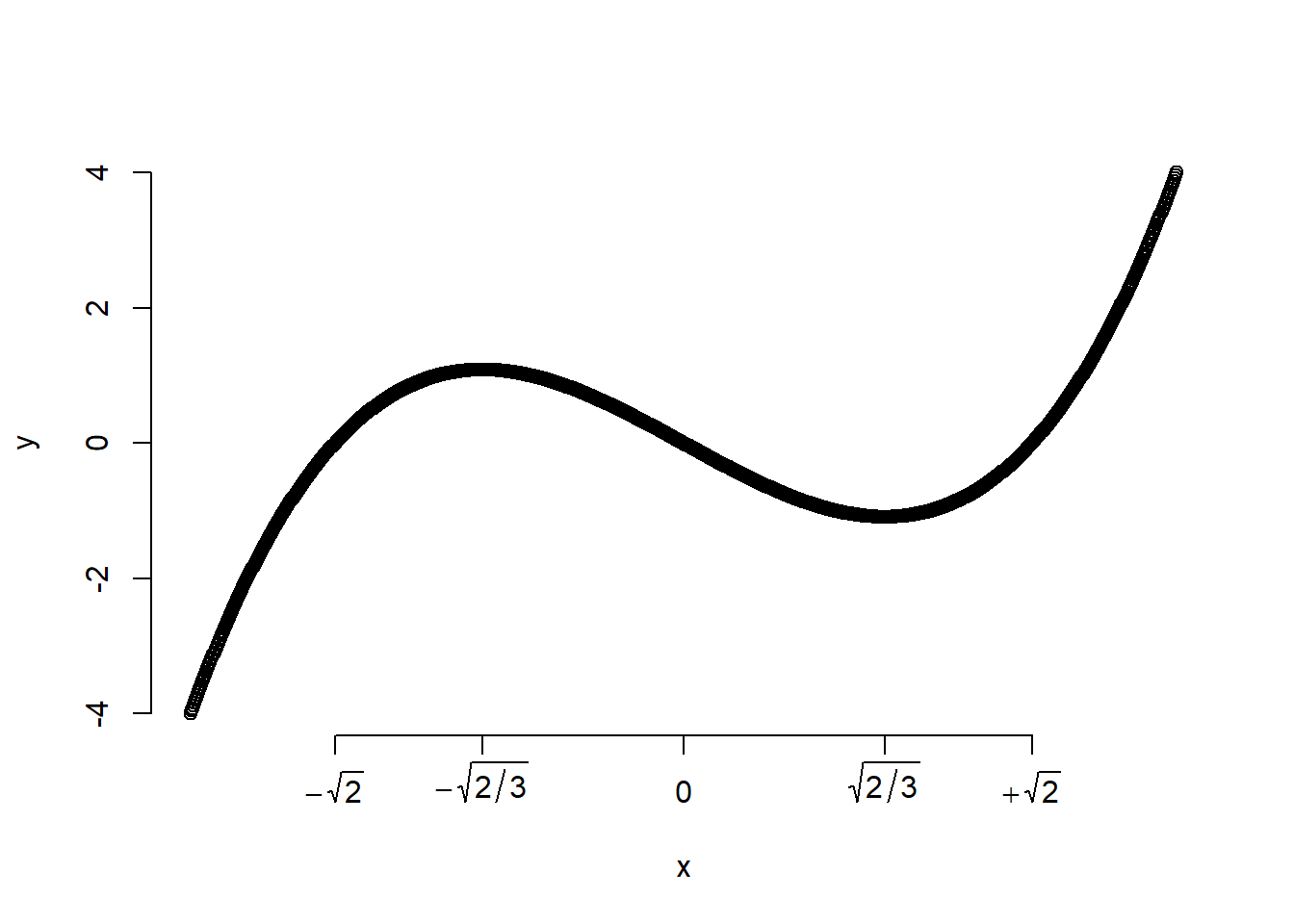Figure 4. Plot with math symbols

You can have the axes cross at the middle. When you do, it no longer makes sense to have a tick mark at zero.

xticks <- c(-sqrt(2),-sqrt(2/3),sqrt(2/3),sqrt(2))
xlabs <- c(expression(-sqrt(2)),expression(-sqrt(2/3)),expression(sqrt(2/3)),expression(+sqrt(2)))
plot(x,y,axes=FALSE)
axis(side=2,pos=0,at=c(-4,-2,2,4))
axis(side=1,at=xticks,labels=xlabs,pos=0)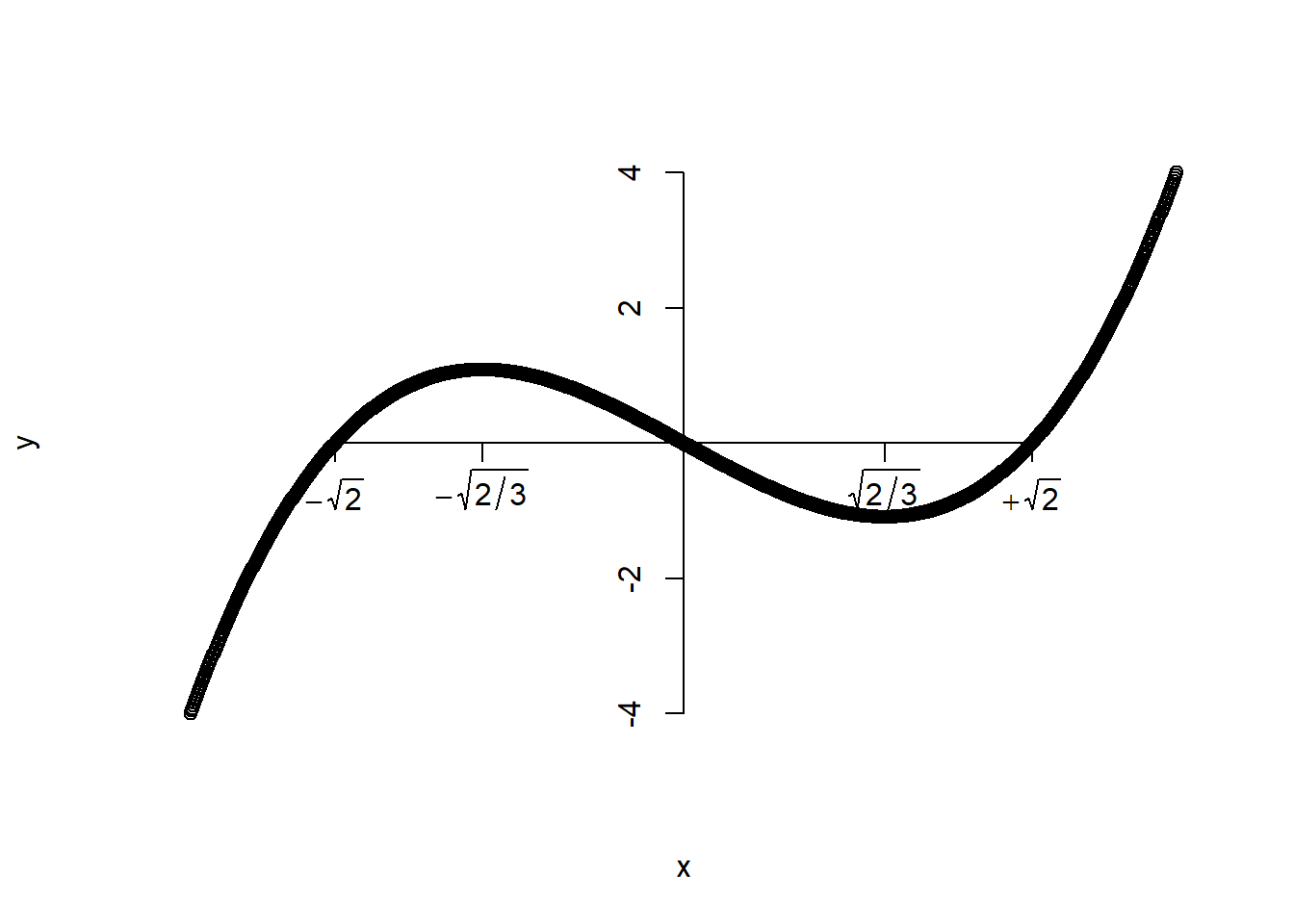Figure 5. Plot with crossed axes.

One important axis option is set in the plot function itself. You are able to put different labels on the x and y axes with the xlab and ylab arguments.

plot(x,y,xlab="Abcissa",ylab="Ordinate")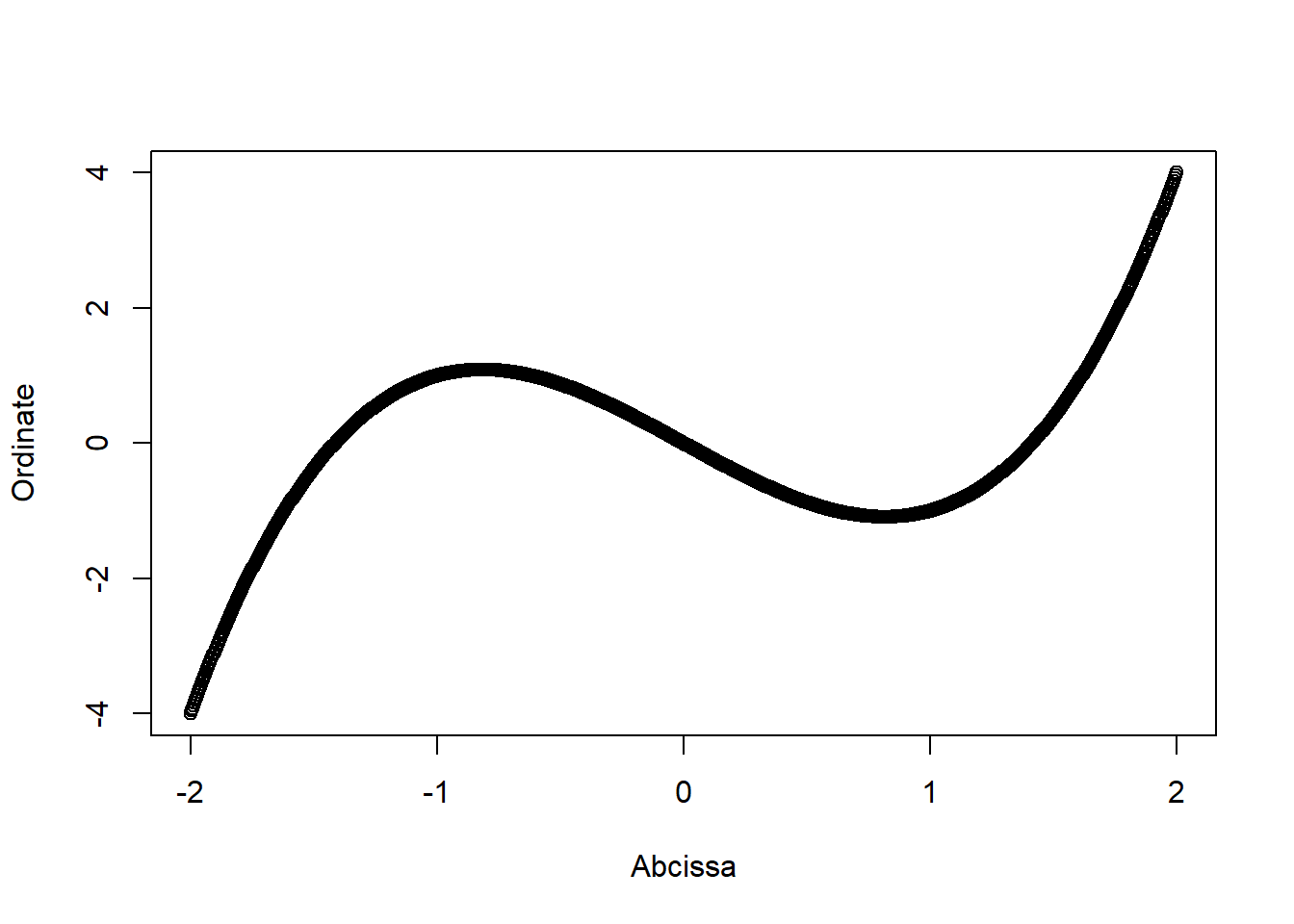Figure 6. Plot with axis labels

Finally, there are several options to control the display of axes that are found in the par function. Here are a couple of the most important ones.

The las argument in par changes the orientation of the tick mark labels.

# las=0 is the default
par(mfrow=c(2,2))
par(las=0)
plot(x,y)
title("las=0: Both parallel")
par(las=1)
plot(x,y)
title("las=1: Both horizontal")
par(las=2)
plot(x,y)
title("las=2: Both perpendicular")
par(las=3)
plot(x,y)
title("las=3: Both vertical")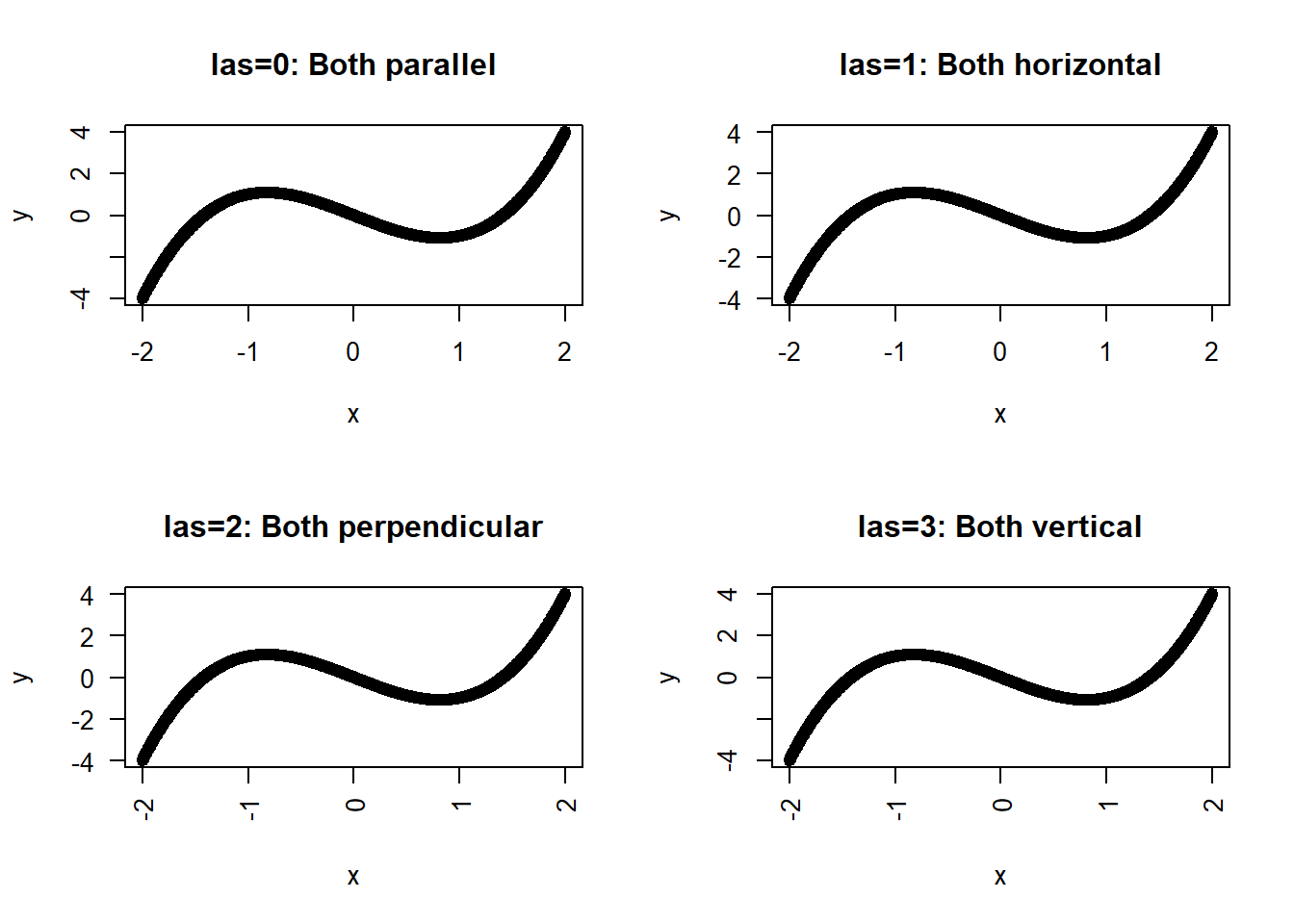Figure 7. Plots with different tick label orientations

The pty argumet in par can also force the plot to be square.

par(pty="s")
plot(x,y)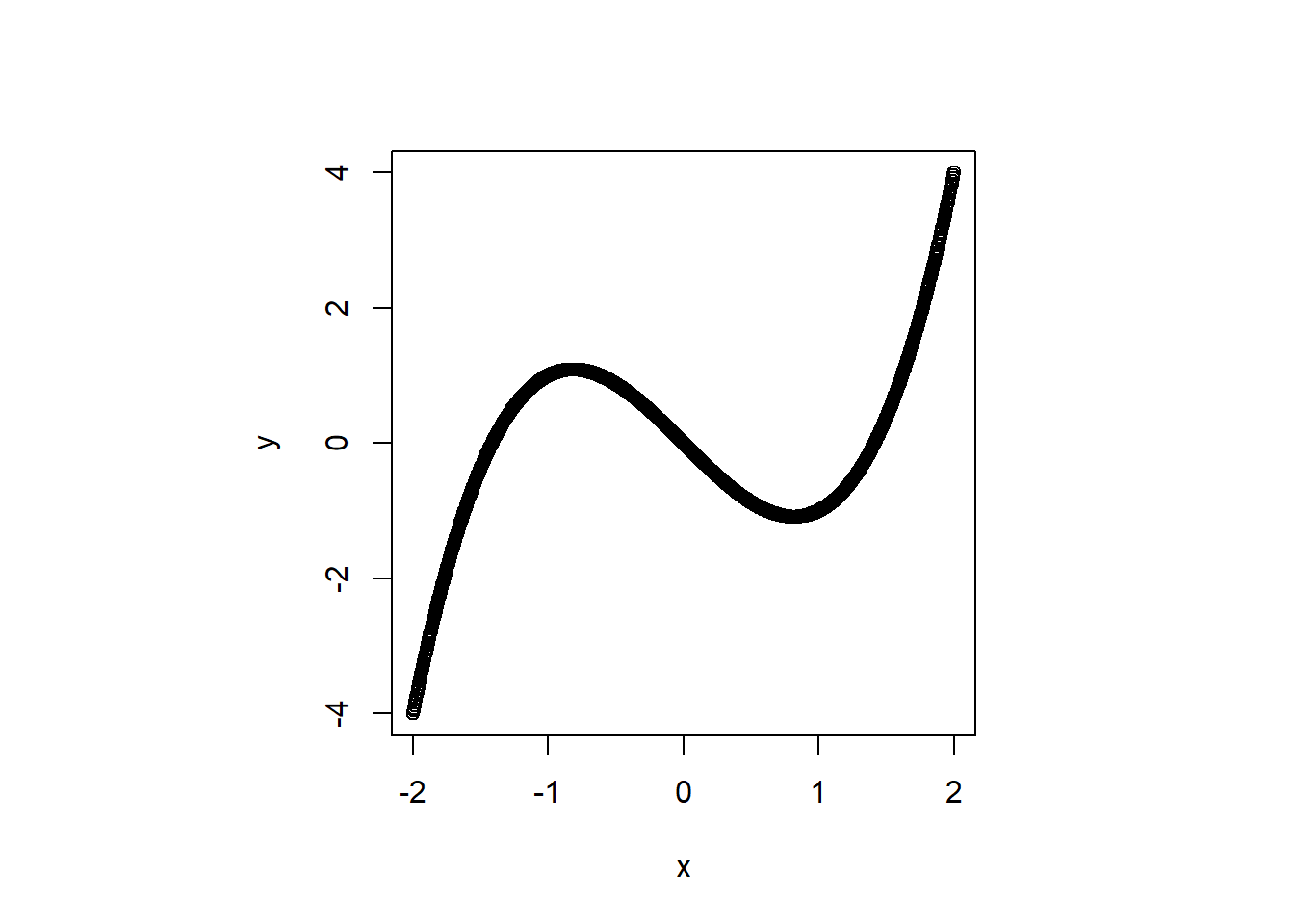Figure 8. Plot with square dimensions

When you combine this with equal axis limits, it creates a graph where the line of unity (y=x) has a 45 degree slope. This is helpful when comparing two measurements on the same units.

par(pty="s")
plot(x,y,xlim=c(-4,4),ylim=c(-4,4))
abline(a=0,b=1)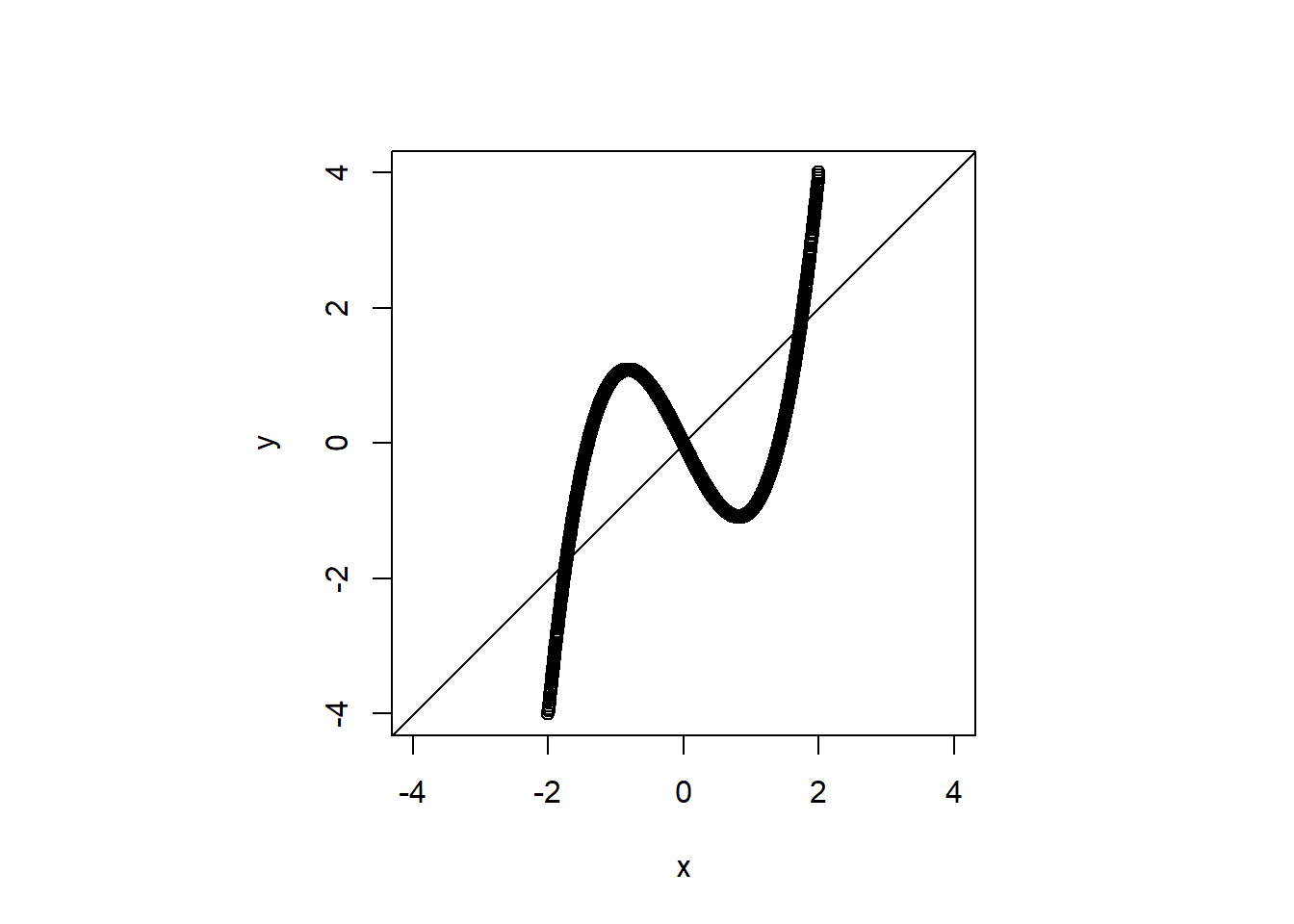Figure 9. Plot with square dimensions and equal scales

You can find an earlier version of this page on my old website.